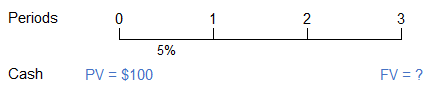# Time Value of Money Calculator

Created by Tibor Pal, PhD candidate
Reviewed by Bogna Szyk and Jack Bowater
Last updated: Feb 27, 2022

The time value of money calculator (TVM) is a simple tool that helps you to find out the future value of a current amount of money. Alternatively, you can use this TVM calculator to compute the present value of money to be received in the future. If you read further, you can learn what is the time value of money definition as well as the time value of money formula, to use with your own computations. If you're managing a project with a monetary budget, check out the expected monetary value calculator that helps you determine how much you need to set aside as contingency for your budget.

## Time value of money definition - what is time value of money (TVM)

The concept of the time value of money is simple: money that you receive now is worth more than the same amount of money in the future since today's money can earn interest between now and then.

You may phrase the time value of money definition more formally; that money obtained at present has a greater advantage over the identical sum in the future due to its potential earning capacity.

You can find the concept of time value analysis behind many financial products, including retirement planning, loan payment schedules, and investment decisions. Undoubtedly, among all concepts applied in finance, the most relevant one is the time value of money (TVM), also called discounted cash flow (DCF) analysis.

Indeed, this approach is the basis for many of our calculators as well; for example, it's been applied in our present value of annuity calculator and in the future value of annuity calculator.

To visualize the problem, let's take a simple example and illustrate it on a timeline. Let's assume you receive $100 today, and you would like to know what this money will be worth in three years.The intervals from 0 to 1, 1 to 2, and 2 to 3 are periods, in our case years. Time 0 is the present, and it is the beginning of Period 1; Time 1 is one year from now, and it is both the end of the Period 1 and the beginning of the Period 2; and so on. The interest rate is 5% (at all intervals); a single cash flow,$100, is invested at Time 0; and the Time 3 value is what we would like to know.

In the following section, you can learn what formula you would need to apply to compute the value in question and how to use our time value of money calculator.

## Time value of money formula - how to use this TVM calculator?

Now that you are familiar with the concept of time value, let's see how you can utilize time value of money calculator to define the future value of present money or the present value of money received in the future.

Before all of that, let's check what parameters you can set during the computation.

• Present value (PV) is the present value of the future money.

• Future value (FV) is the future value of the present amount.

• Interest rate (i) is the annual nominal interest rate per period in percent.

• Term (t) constitutes the lifespan between the present (Time 0) and the future time we are calculating to (Time x), converted into years.

• Compounding frequency (n) refers to the number of times compounding occurs per period. You can choose the frequency as continuous as well, which is theoretically the maximum compounding frequency. In this case, the number of periods when compounding applies is an infinite number.

This calculator works in such a way that you can input your known values and you will receive the value you want. It's that simple!

Finally, the time value of money formulas employed during the computation are the following:

FV = (PV * (1 + (i / n)) ^ (n * t))

PV = (FV / (1 + (i / n)) ^ (n * t))

In the case of continuous compounding, the below equations are used:

FV = PV * e ^ (i * t)

PV = FV / e ^ (i * t)

where e stands for the exponential constant, which is approximately 2.718.

Now, we can easily estimate the future value of $100 from the previously mentioned simple example. PV = 100$

t = 3

i = 5%

n = 1

FV = (100 * (1 + (5 / 1)) ^ (1 * 3)) = 115.76

Thus, $100 in your pocket now would worth$115.76 three years later if a 5 percent interest rate is applied and compounding occurs yearly.

• Eugene F. Brigham and Michael C. Ehrhardt: Financial Management - Theory & Practice (15e) - 2017, Cengage Learning
Tibor Pal, PhD candidate
Interest rate
%
Term
yrs
mos
Compound frequency
Yearly
Present value
$Future value$
People also viewed…

### Finance charge

Use the finance charge calculator to estimate the cost imposed on your credit balance in a given billing cycle.

### Plastic Footprint

Find out how much plastic you use throughout a year with this plastic footprint calculator. Rethink your habits, reduce your plastic waste, and make your life a little greener.